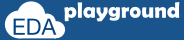# priority if

Priority if evaluates all the conditions in sequential order.

In the following conditions simulator issue a run time error/warning
• No condition is true or final if doesn't have corresponding else

## priority if examples

In the below example,
No condition is true or final if doesn't have corresponding else.
value of a=50,b=20 and c=40. conditions a<b and a<c are false,

therefore simulator issue a run time warning.
"RT Warning: No condition matches in 'priority if' statement."

```module priority_if;
```
```  //variables declaration
int a,b,c;
```
```   initial begin
//initialization
a=50;
b=20;
c=40;

priority if ( a < b ) \$display("\t a is less than b");
else     if ( a < c ) \$display("\t a is less than c");
end```
` endmodule`

Simulator output:

RT Warning: No condition matches in 'priority if' statement.

Execute the above code onExample-2:

In the below example,
value of a=10,b=20 and c=40.
conditions a<b and a<c are true, as it is priority based, simulator
considers the first match. therefore there will be no simulator warning message.

```module priority_if;

//variables declaration
int a,b,c;

initial begin
//initialization
a=10;
b=20;
c=40;

priority if ( a < b ) \$display("\t a is less than b");
else     if ( a < c ) \$display("\t a is less than c");
else                \$display("\t a is greater than b and c");
end

endmodule```

Simulator output:

a is less than b

Execute the above code on